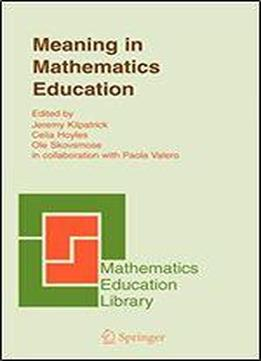# Meaning In Mathematics Education by Jeremy Kilpatrick / 2005 / English / PDF

What does it mean to know mathematics? How does meaning in mathematics education connect to common sense or to the meaning of mathematics itself? How are meanings constructed and communicated and what are the dilemmas related to these processes? There are many answers to these questions, some of which might appear to be contradictory. Thus understanding the complexity of meaning in mathematics education is a matter of huge importance. There are twin directions in which discussions have developedtheoretical and practicaland this book seeks to move the debate forward along both dimensions while seeking to relate them where appropriate. A discussion of meaning can start from a theoretical examination of mathematics and how mathematicians over time have made sense of their work. However, from a more practical perspective, anybody involved in teaching mathematics is faced with the need to orchestrate the myriad of meanings derived from multiple sources that students develop of mathematical knowledge. This book presents a wide variety of theoretical reflections and research results about meaning in mathematics and mathematics education based on long-term and collective reflection by the group of authors as a whole. It is the outcome of the work of the BACOMET (BAsic COmponents of Mathematics Education for Teachers) group who spent several years deliberating on this topic. The ten chapters in this book, both separately and together, provide a substantial contribution to clarifying the complex issue of meaning in mathematics education. This book is of interest to researchers in mathematics education, graduate students of mathematics education, under graduate students in mathematics, secondary mathematics teachers and primary teachers with an interest in mathematics.

views: 383